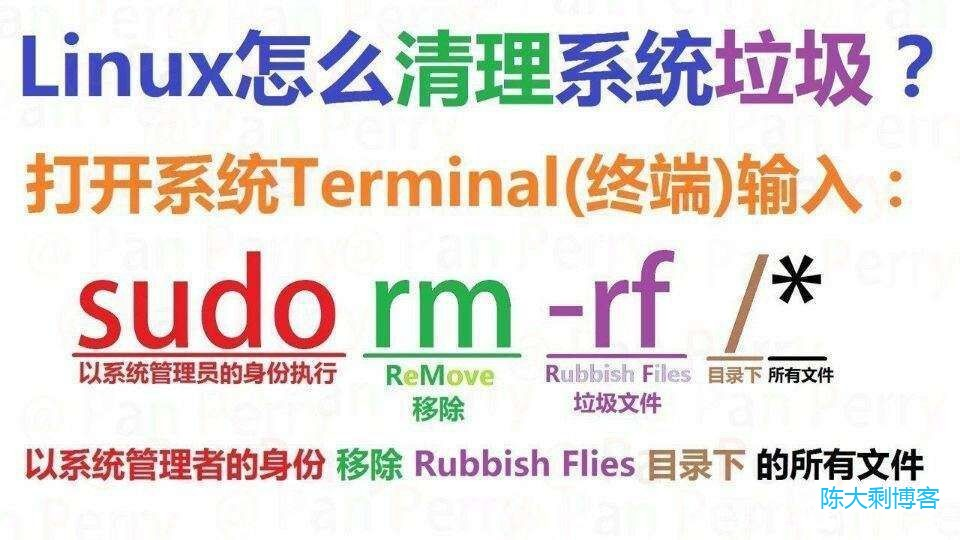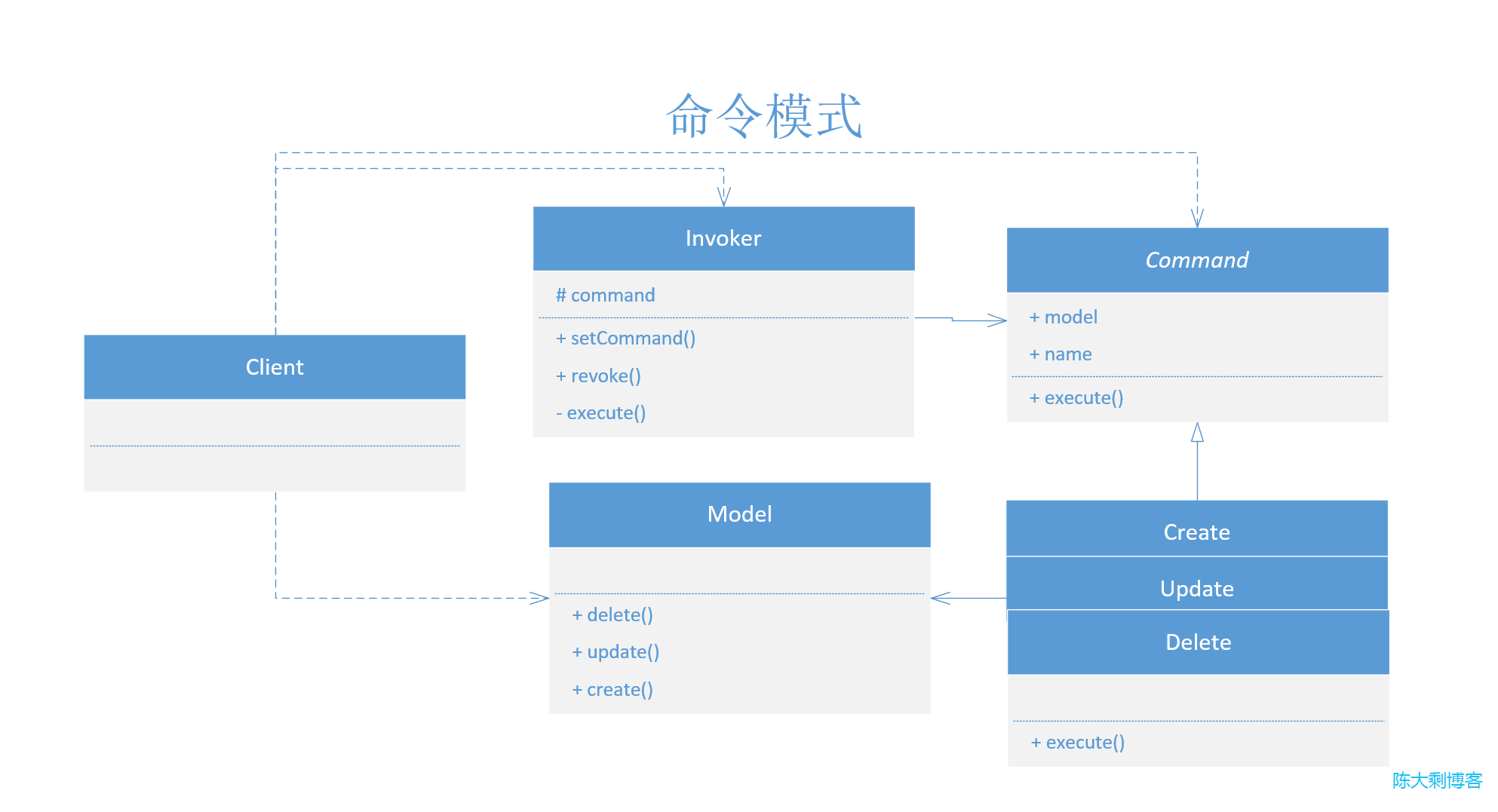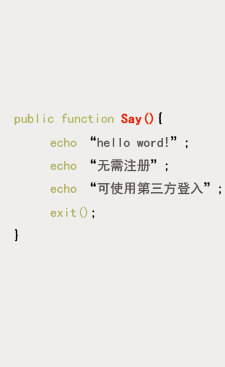# php设计模式(十六)：命令模式（Command）

• 陈大剩
• 2023-05-11 17:34:11
• 53

## 问题## UML## 结构

Command：命令抽象类；一般会暴露一个 execute 方法；
Delete、Create、Update：具体的命令类；设定接收者；
Invoker：命令发送者；用于调用命令；
Model： 命令接收者；负责执行操作；

## 代码示例

### 命令接口基类

/**
* 命令模式接口基类
*/
abstract class Command
{
/**
* 模型
* @var Model
*/
public Model $model; /** * 命令名称 * @var */ public$name;

/**
* 执行方法
* @return mixed
* @author chendashengpc
*/
abstract public function execute();

}


### 命令具体类

/**
* 删除
*/
class Delete extends Command
{
/**
* 初始化函数
* @param Model $model */ public function __construct(Model$model)
{
$this->name = 'delete';$this->model = $model; } /** * 执行函数 * @return mixed|void * @author chendashengpc */ public function execute() { return$this->model->delete();
}
}


/**
* 创建
*/
class Create extends Command
{
/**
* 初始化函数
* @param Model $model */ public function __construct(Model$model)
{
$this->name = 'create';$this->model = $model; } /** * 执行函数 * @return mixed|void * @author chendashengpc */ public function execute() { return$this->model->create();
}


/**
* 更新
*/
class Update extends Command
{
/**
* 初始化函数
* @param Model $model */ public function __construct(Model$model)
{
$this->name = 'update';$this->model = $model; } /** * 执行函数 * @return mixed|void * @author chendashengpc */ public function execute() { return$this->model->update();
}
}


### 调用类

/**
* 调用类
*/
class Invoker
{
protected $command = []; /** * 设置命令 * @param Command$command
* @return void
* @author chendashengpc
*/
public function setCommand(Command $command) {$this->command[$command->name] =$command;
}

/**
* 撤销命令
* @return void
* @author chendashengpc
*/
public function revoke(Command $command) { if (isset($this->command[$command->name])) { unset($this->command[$command->name]); } } /** * 执行命令 * @return void * @author chendashengpc */ public function execute() { foreach ($this->command as $command) { echo$command->execute() . PHP_EOL;
}
}
}


### 具体接受者类（Model）

/**
* 具体接受者类（Model类）
*/
class Model
{

/**
* 删除
* @return void
* @author chendashengpc
*/
public function delete()
{
return '删除命令';
}

/**
* 更新
* @return void
* @author chendashengpc
*/
public function update()
{
return '更新命令';
}

/**
* 新增
* @return string
* @author chendashengpc
*/
public function create()
{
return '新增命令';
}
}


### 客户端使用

// 新建一个模型
$model = new Model();$create = new Create($model);$update = new Update($model);$delete = new Delete($model); // 设置一个调用者$invoker = new Invoker();
$invoker->setCommand($create);
$invoker->setCommand($update);
$invoker->setCommand($delete);
$invoker->revoke($delete);

\$invoker->execute();


新增命令



## 优缺点

### 优点

• 单一职责原则。 可以解耦触发和执行操作的类。
• 开闭原则。 可以在不修改已有客户端代码的情况下在程序 中创建新的命令。
• 可以实现撤销和恢复功能。
• 可以实现操作的延迟执行。
• 可以将一组简单命令组合成一个复杂命令。

### 缺点

• 代码可能会变得更加复杂，因为在发送者和接收者之间增 加了一个全新的层次。

0

## 说点儿什么吧表情

## 留言墙